Like   Pin

## Thursday, July 25, 2019

 table div table+table+table div table{width:100%;padding:0}table div table+table+table div table img{width:96.23%;padding:0;float:none}table div table+table+table div table td{width:100%;padding:0 1.88% 18px}/* styles */# Sunday's Service - July 28, 2019

 /* styles */ We welcome back Adam Shank to lead us in Worship this week. The featured Scripture verses are: 1 Peter 1:22 – 2:3 for Adam's message: As Newborn Babes. We will celebrate the Sacrament of Communion.
 /* styles */ ~~~

# CG NEWS & EVENTS## Bible Studies

Sunday Morning Class: Romans, 9:30a.m.
Monday Morning Ladies Study: Hosea, 9:30am

You are welcome to join in studies at any time!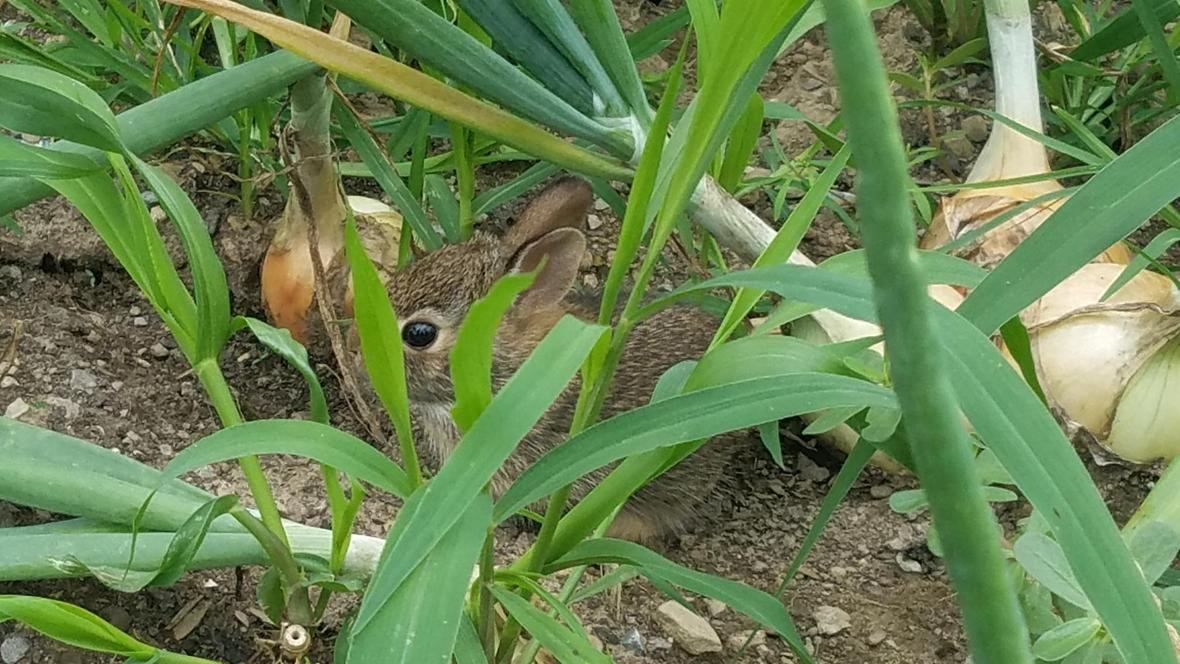## Scenes From Last Sunday

Thanks to Darius for joining Jess to lead singing last week! Thanks also go to Mia and Liam for being such good sports and participating in a "Children's Sermon." We always appreciate these young people!
We enjoyed a visit to the raised bed garden to see our onion bed bunnies, who have since hopped on out and headed off into the world.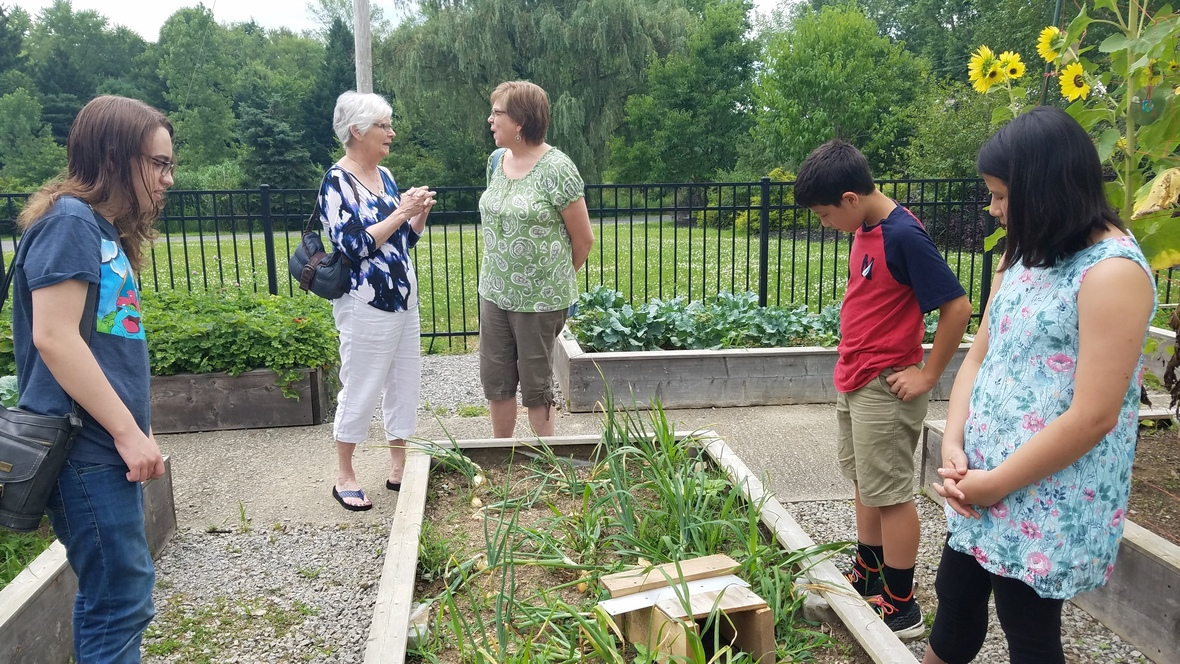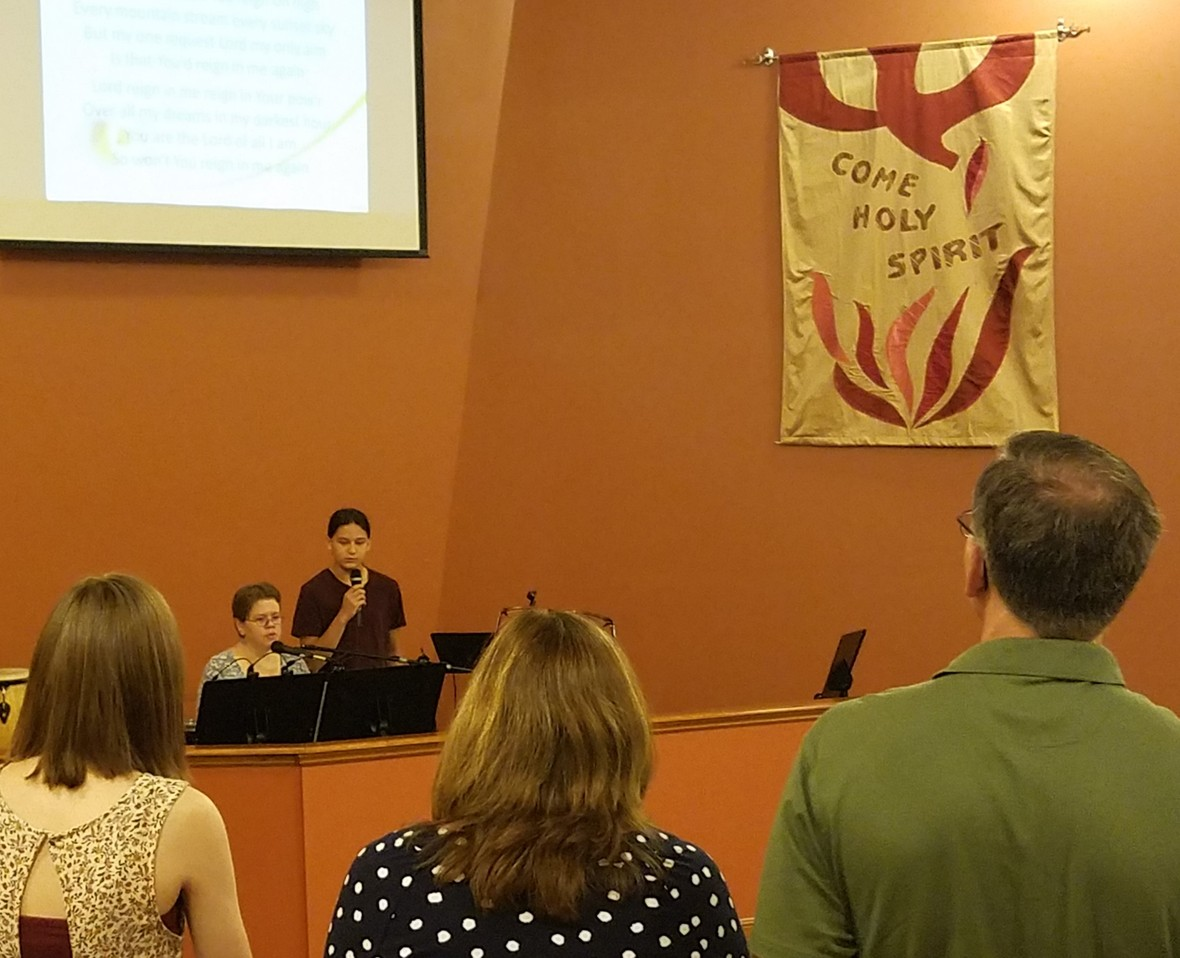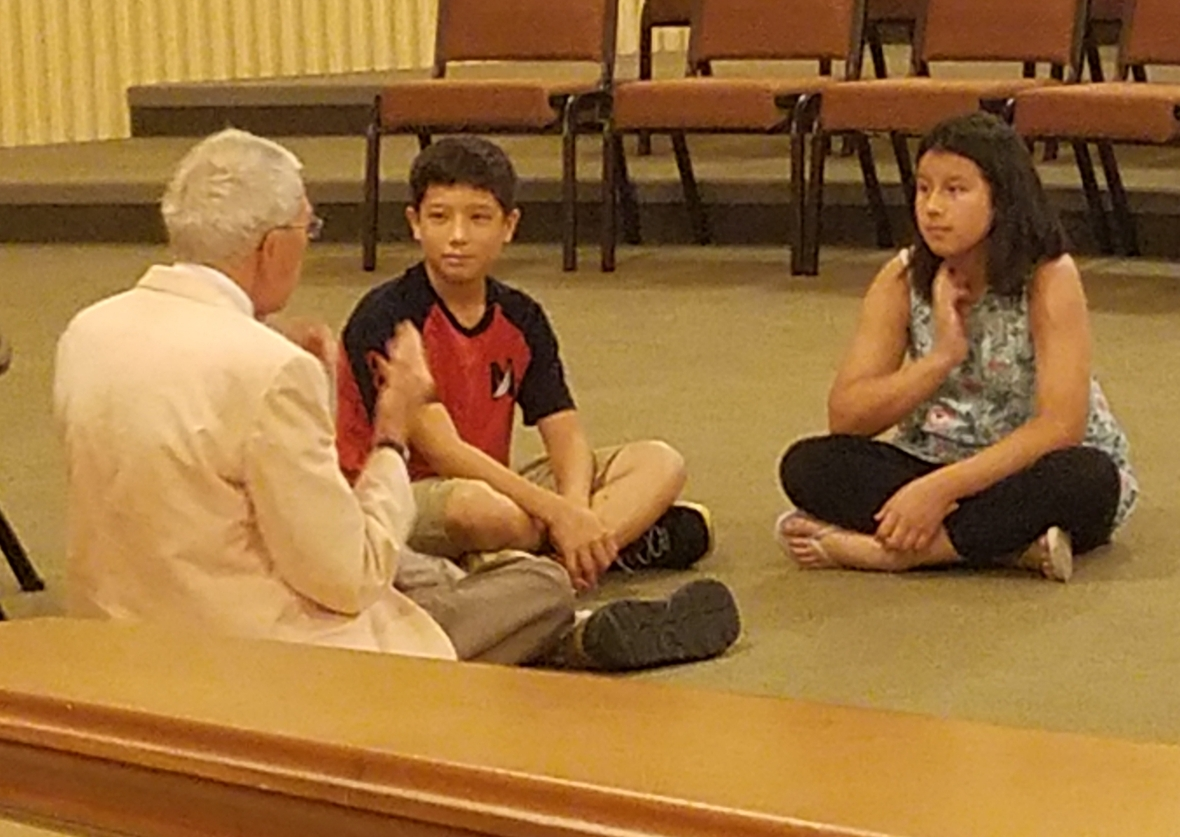## Garden & Nursery News

The Garden is on Summer Break until September 8th. Activity pages will be on the table by the Sanctuary doors. Children 3 & younger are invited to the Nursery for Bible story time.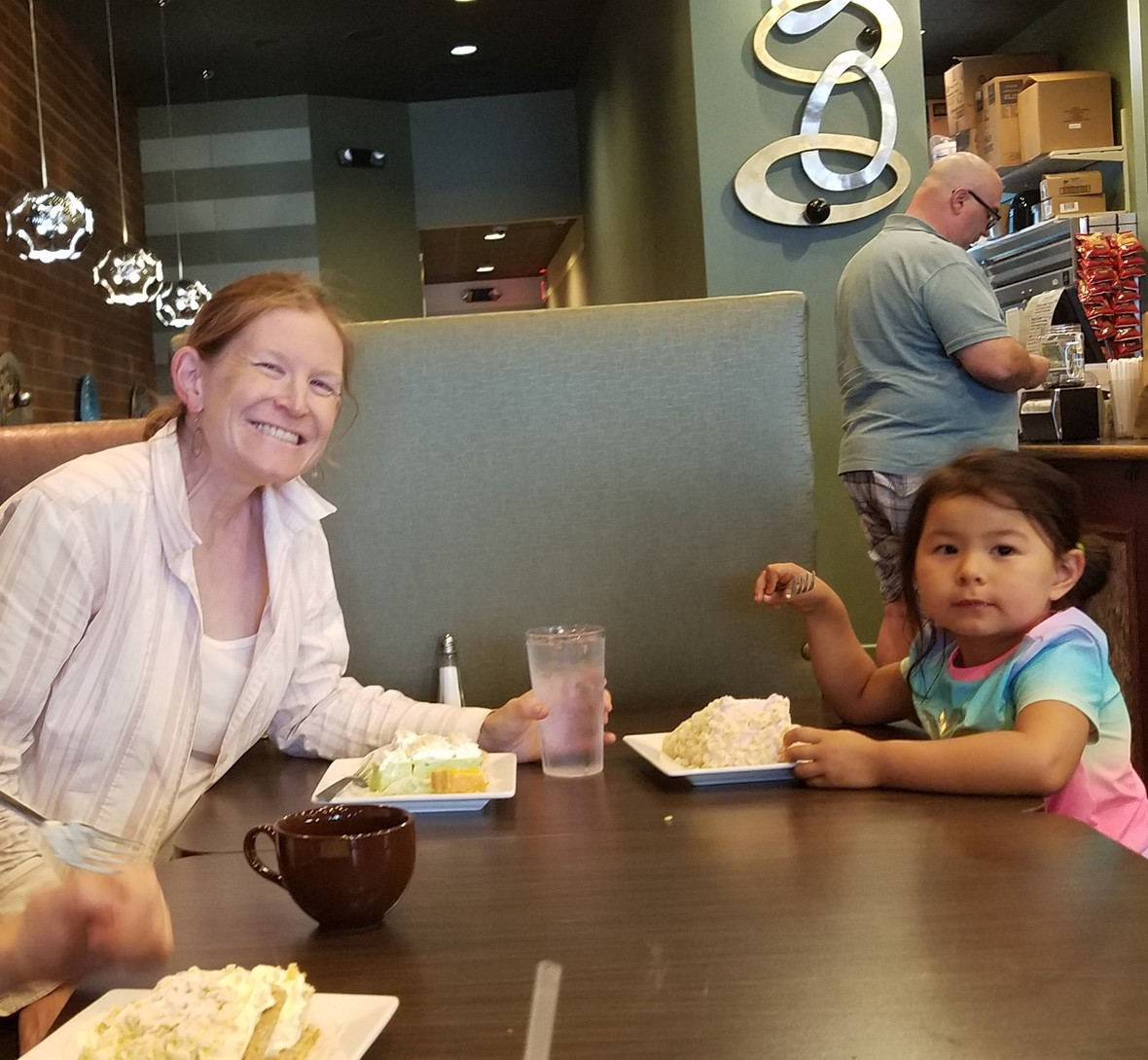## Old Friends Visited

Take a look at who was in town this weekend! Our very own Miss Meagan and her daughter, Lindy! Lindy enjoyed a stop in Boardman to celebrate her 4th birthday with some incredible desserts.## Youth Group

All youth who will be entering 5th grade and up this fall
are invited to join in the fun this summer!

▪ Lock In THIS WEEKEND! ~ Sunday, July 28, 6pm - Monday, July 29, 8am This year we have changed the day of the week and will start by cooking dinner together! Participants need to bring a snack to share, a flashlight, and whatever they need for an overnight stay. We will (hopefully) have some outside activities, so please wear sneakers - NOT flip flops/slides. You may also want to send bug spray. Please sign up or contact Jen L. by this evening.
 ▪ Lock In THIS WEEKEND! ~ Sunday, July 28, 6pm - Monday, July 29, 8am This year we have changed the day of the week and will start by cooking dinner together! Participants need to bring a snack to share, a flashlight, and whatever they need for an overnight stay. We will (hopefully) have some outside activities, so please wear sneakers - NOT flip flops/slides. You may also want to send bug spray. Please sign up or contact Jen L. by this evening.

## Fun at Last Year's Lock In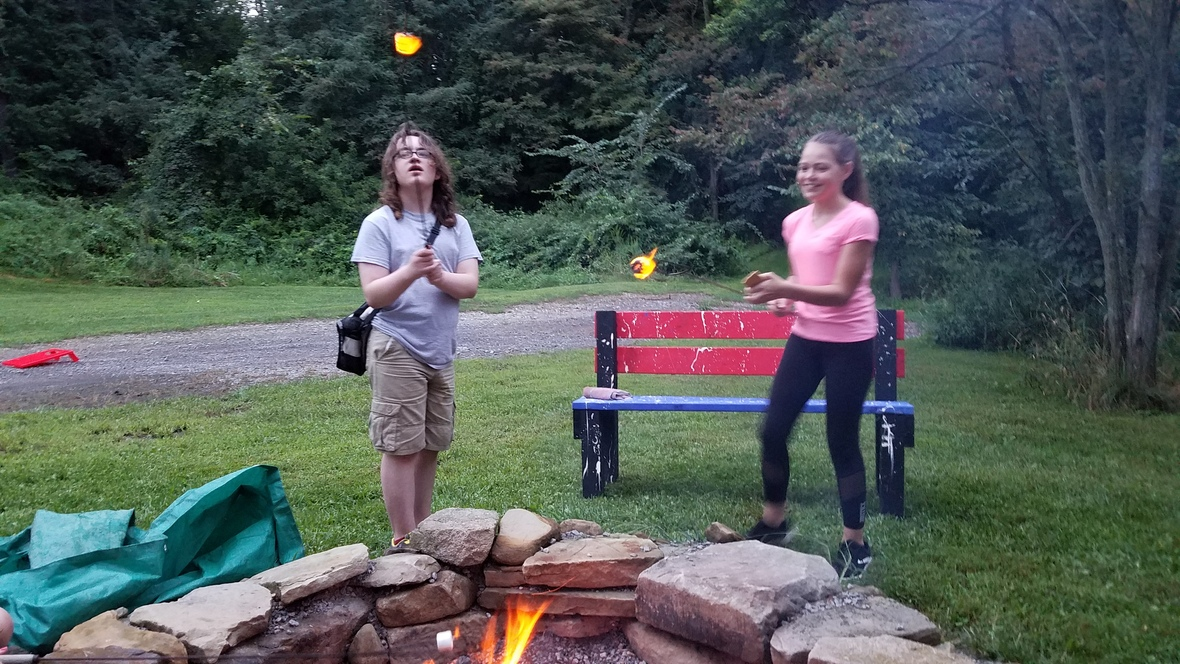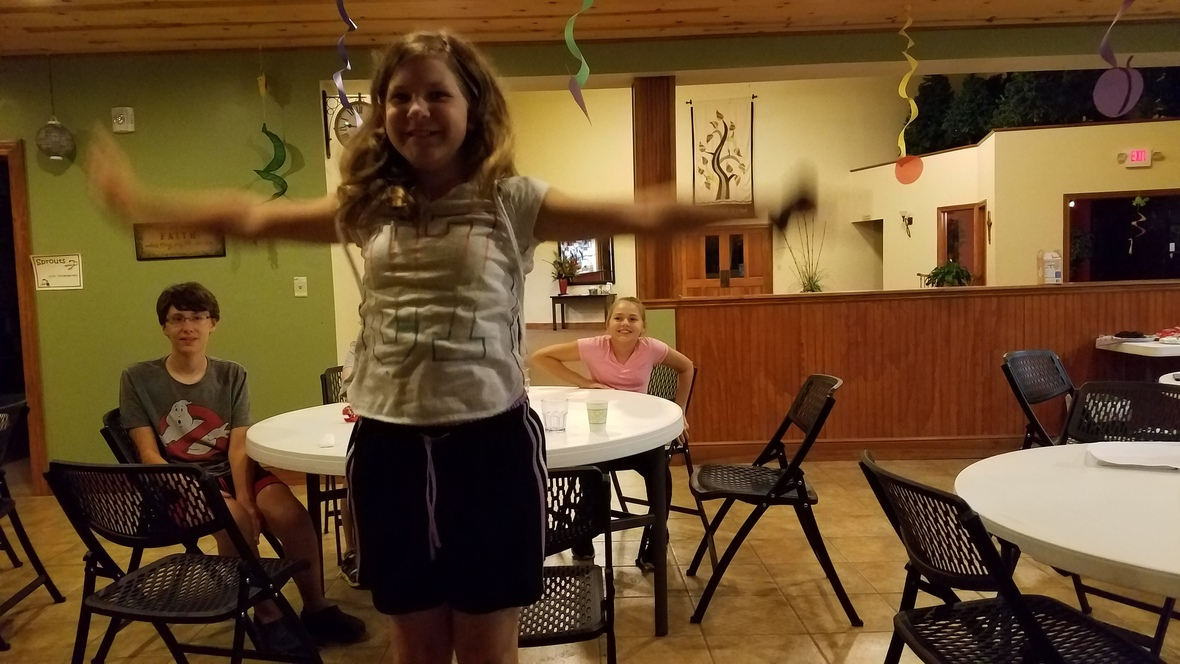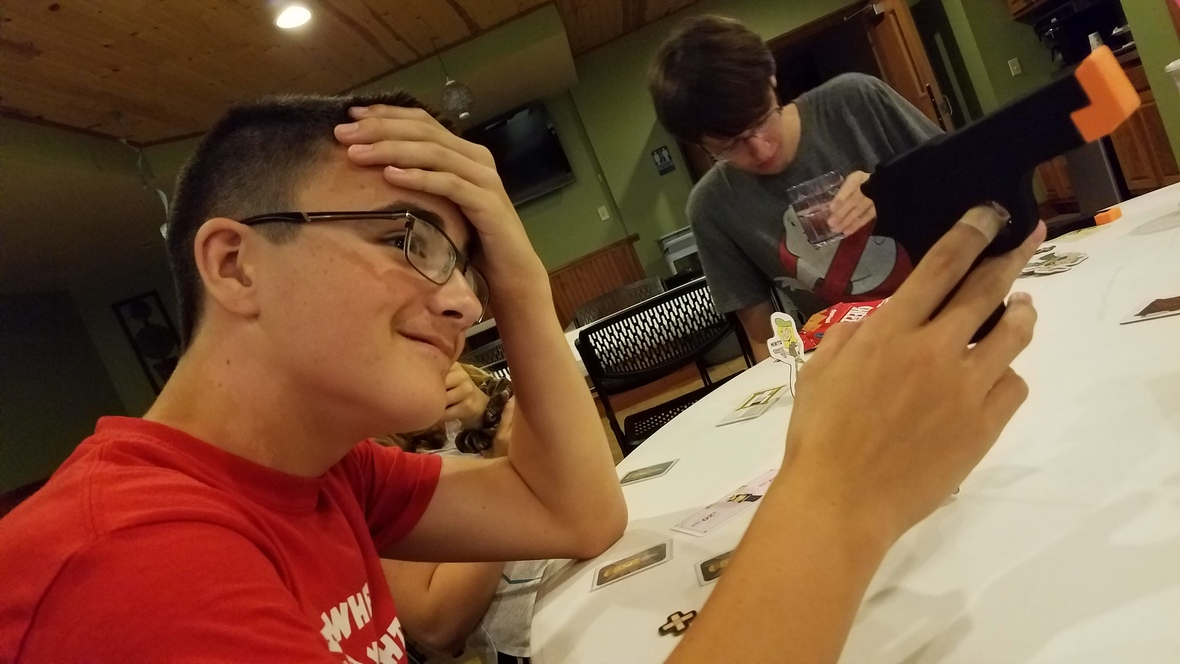▪ End of Summer Party at the Vignon's ~ Friday, August 16, 6pm Bonfire and hot dogs to get ready to head back to school!
 ▪ End of Summer Party at the Vignon's ~ Friday, August 16, 6pm Bonfire and hot dogs to get ready to head back to school!
 table div table+table+table+table+table+table+table+table+table+table+table+table+table+table+table+table+table+table div table{width:100%;padding:0}table div table+table+table+table+table+table+table+table+table+table+table+table+table+table+table+table+table+table div table img{width:96.23%;padding:0;float:none}table div table+table+table+table+table+table+table+table+table+table+table+table+table+table+table+table+table+table div table td{width:100%;padding:0 1.88% 18px}/* styles */# UPCOMING AT CG## August Speakers

4 - John Muckridge
11 - Steve Scott
18 -
25 - Phil Bartholomew

## Spaghetti Sauce Canning Class

Saturday, August 24, 10am-2pm (or until we're done)
Sign up on the bulletin board to join us for this tomato extravaganza with Rachel Vignon. She is willing to share her family recipe and teach us all how to make a truly delicious spaghetti sauce. Jars will be provided. There will be a small fee to cover ingredients. (Fee - around \$5 or less - to be determined when we have a total count of participants.) The ending time of 2pm is really just a guess. We could be done much faster or a little later...depending on our kitchen skills and number of participants. But, no matter how long it takes, you won't regret this "summer school" opportunity!

 table div table+table+table+table+table+table+table+table+table+table+table+table+table+table+table+table+table+table+table+table+table+table div table{width:100%;padding:0}table div table+table+table+table+table+table+table+table+table+table+table+table+table+table+table+table+table+table+table+table+table+table div table img{width:96.23%;padding:0;float:none}table div table+table+table+table+table+table+table+table+table+table+table+table+table+table+table+table+table+table+table+table+table+table div table td{width:100%;padding:0 1.88% 18px}/* styles *//* styles */ www.goodnessgrows4all.org Our Mission Statement: To cultivate personal, social, and community growth through gardening, education, and opportunity for people of all abilities. ~~~

# NEWS & EVENTS## Summer at GG

The Mission Group from Uniontown, PA's Trinity United Presbyterian Church joined us Monday. Some of them were also with us last summer and had the experience of working out in the gardens during some very toasty days. This year they worked inside and participated in jam making, tie-dying bags, cleaning up the greenhouse, and, of course, painting a rock for us. Two of the folks also helped Rachel make Communion bread for this Sunday, over which they even said a prayer. So, this Sunday's Communion at Common Ground will include fresh bread blessed by a pastor from Trinity. We thank them for their prayers, hard work, and good humor!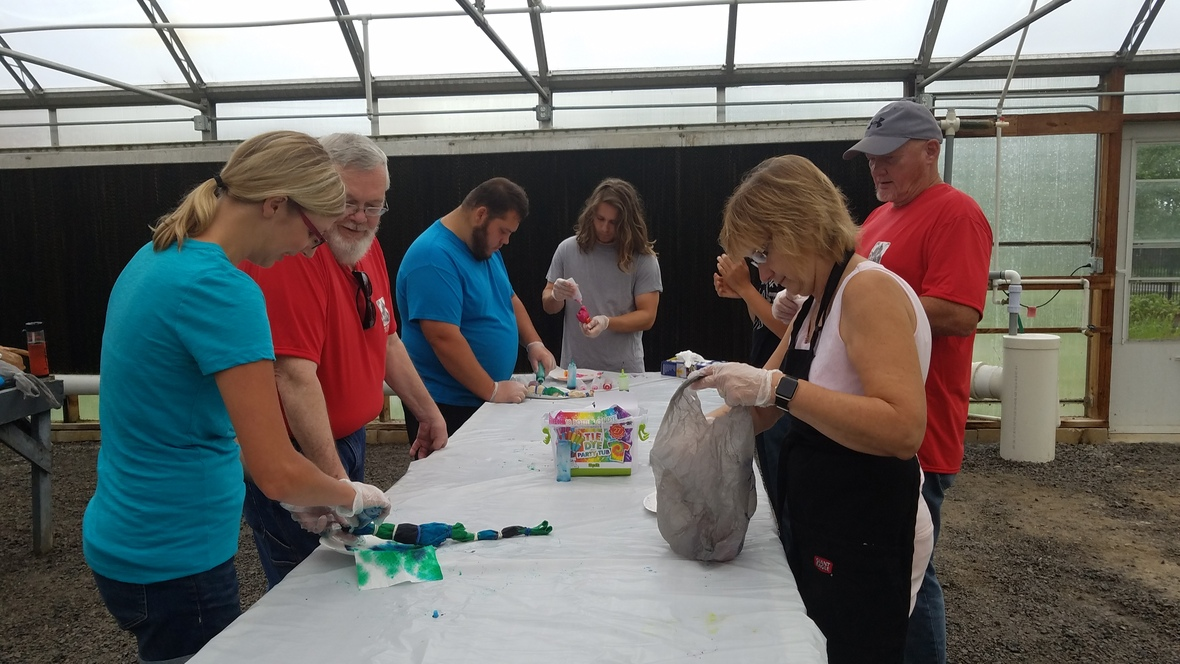Tie-Dye Time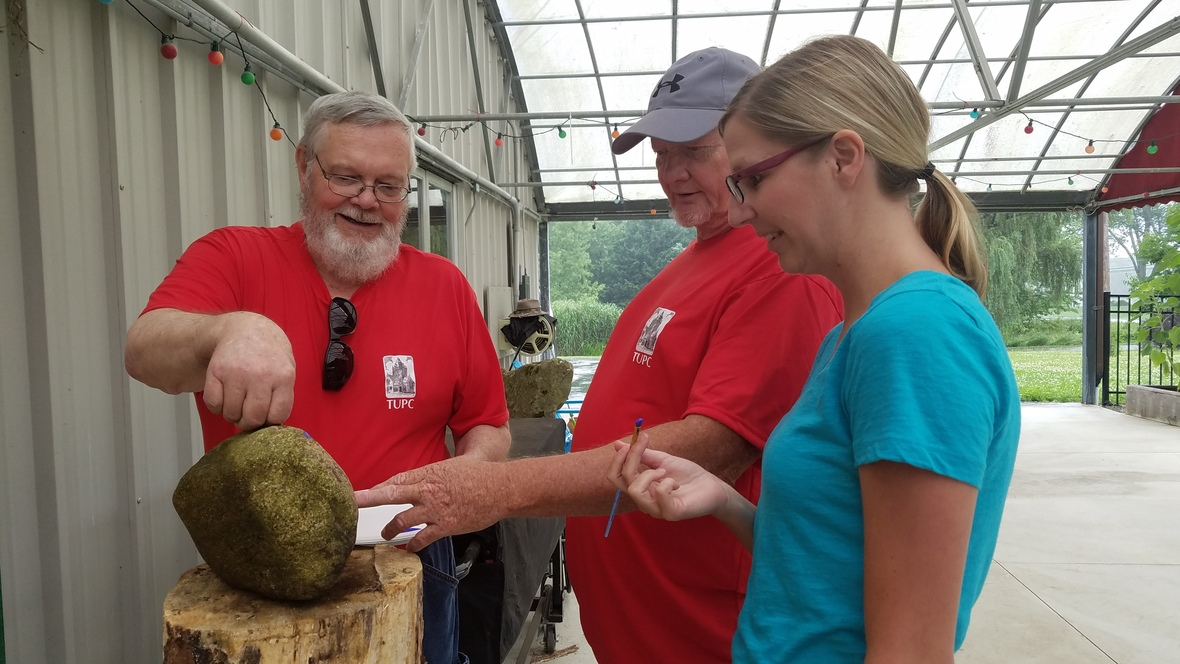Rocking On!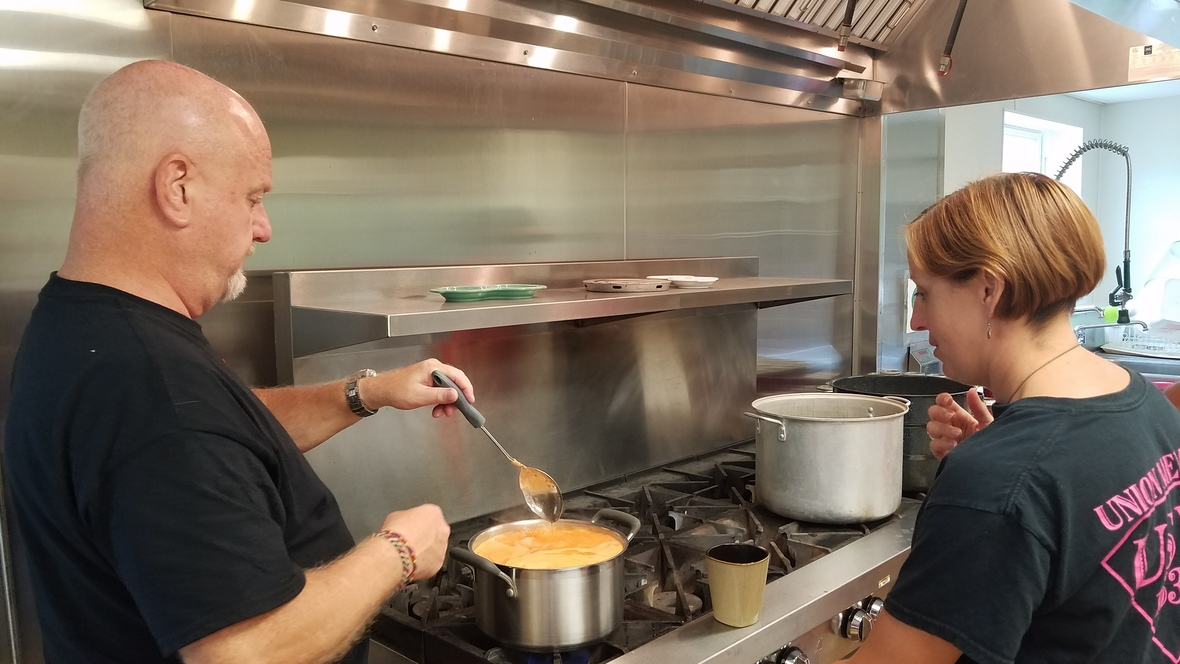Jam Chefs
 /* styles */ Tuesday's feast with Sue found our crew cooking chicken fajitas as well as making some homemade applesauce for next week. They pulled off another culinary delight! The rest of the week was filled with CSA prep...which included making fresh Basil Pesto...cleaning inside, and gardening tasks outside that always need done. There certainly is never a shortage of weeds! Stop by anytime to pull a few while appreciating the wonder of God's beautiful Creation!

## We Are Family

Last Friday we took a field trip to Columbiana for a lunchtime feast at Chang Tai Chinese restaurant, as we bid farewell to one of our interns, Cameron Fortune. He will spend the rest of summer at football practice but we hope he returns next year! We will also soon say good-bye to our second year intern, Darius Surgenavic. In less than three weeks, he will head off to college. We sure were lucky to have him be part of our GG family for two summers...and we'd certainly love to welcome him back next year! Our long-time intern, Trevor Wilson, will thankfully be with us throughout the summer. We are keeping our fingers crossed we don't have to say good-bye to him any time soon!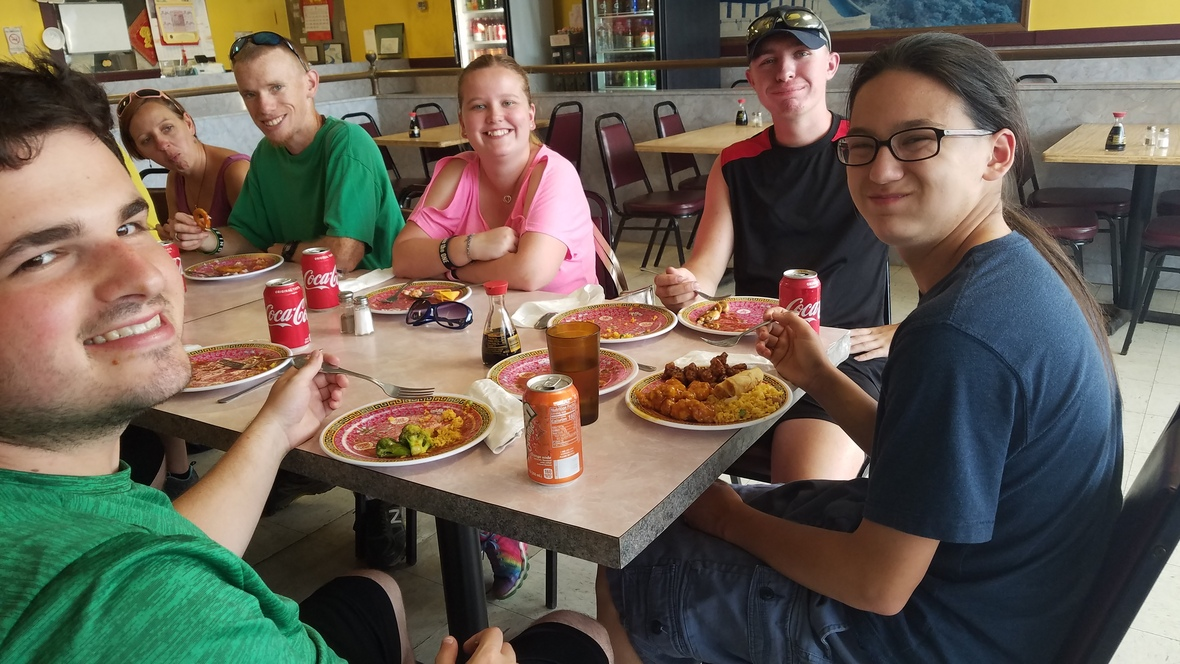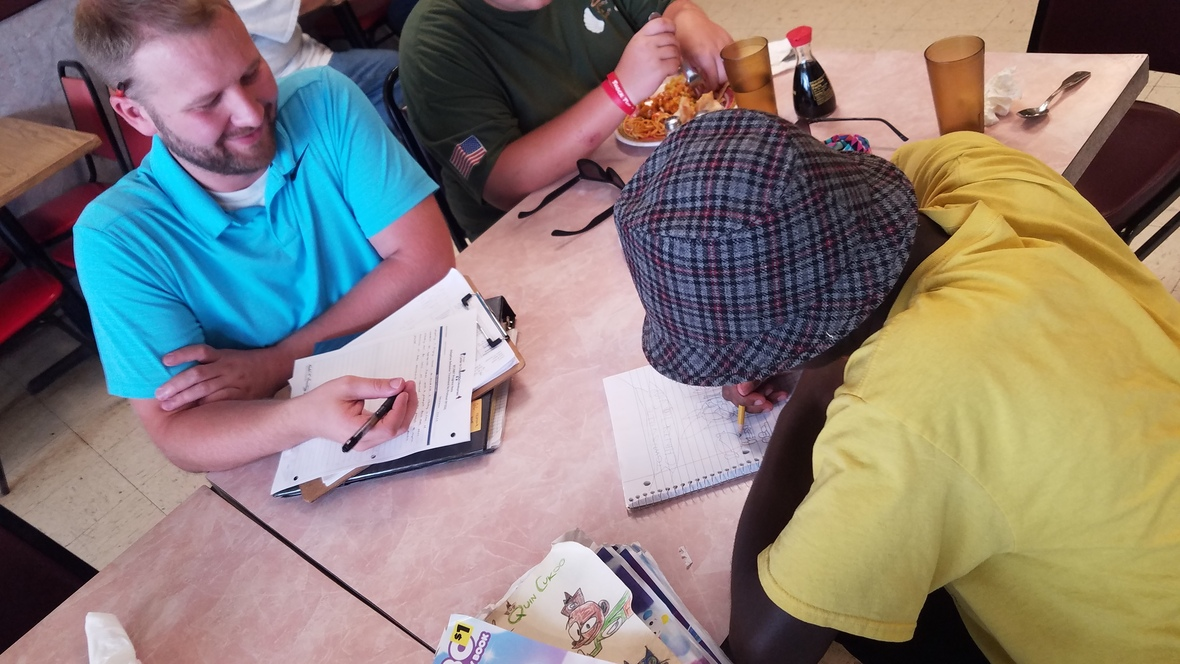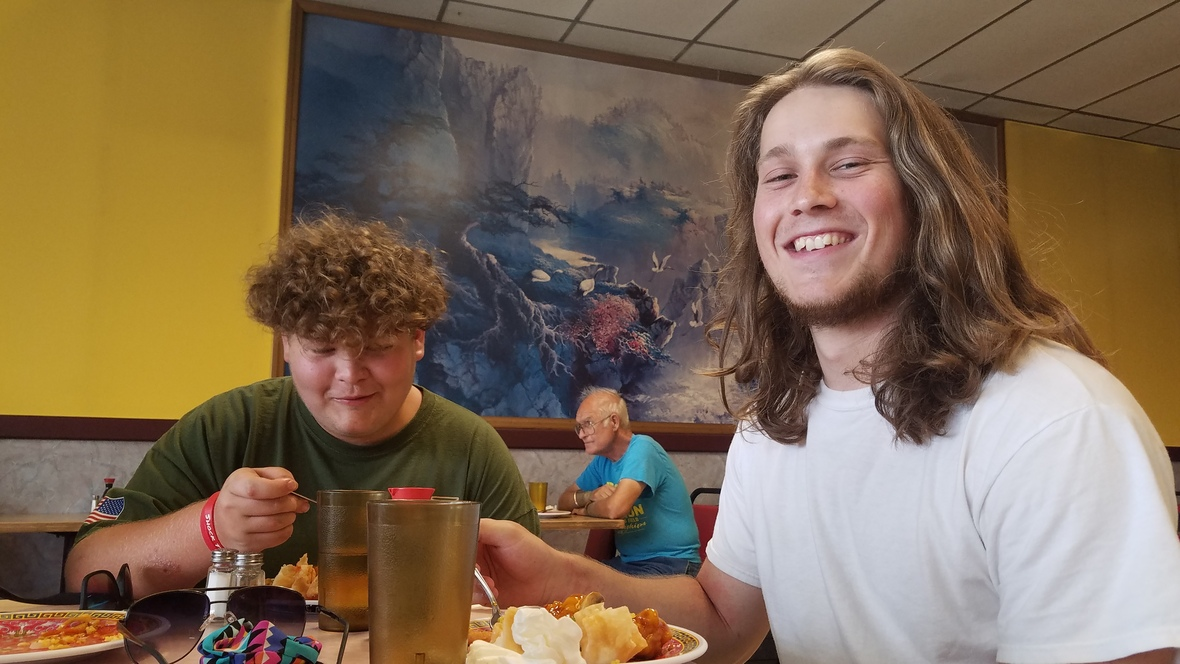## Celebration in Columbiana This Saturday

There will be lots going on this weekend in Columbiana, and GG will be there with Jungle Jam and Juicy Jam from at least 2-6pm. We will be helping to celebrate the food truck A Movable Feast's one year anniversary. They have a big party planned, will be serving some incredible food, and it's all happening right on Main St. near Birdfish! Live music, seating in the Garden of Eatin', yard games, vendors, and more. Come check it out and join in the celebration!

 table div table+table+table+table+table+table+table+table+table+table+table+table+table+table+table+table+table+table+table+table+table+table+table+table+table+table+table+table+table+table+table+table div table{width:100%;padding:0}table div table+table+table+table+table+table+table+table+table+table+table+table+table+table+table+table+table+table+table+table+table+table+table+table+table+table+table+table+table+table+table+table div table img{width:96.23%;padding:0;float:none}table div table+table+table+table+table+table+table+table+table+table+table+table+table+table+table+table+table+table+table+table+table+table+table+table+table+table+table+table+table+table+table+table div table td{width:100%;padding:0 1.88% 18px}/* styles */# OUTREACH and MISSION OPPORTUNITIES## Coat & Clothing Drive

Ongoing Outreach/Mission Opportunity
Drop items off in the collection barrel or take them up to the Green Room, anytime throughout the year! Let your friends and family know that we accept donations year-round. We are happy to take coats, clothes, shoes, purses and bags, jewelry, hats, gloves and mittens, scarves, and even small household items. Anything that is clean and in good repair that you think someone else might be able to use!

Date for Giveaway Set ~ Saturday, October 12th, 10am-1pm!

 /* styles */ ~~
 /* styles */ For I was hungry and you gave me food; I was thirsty and you gave me a drink; I was a stranger and you took me in; I was naked and you clothed me; I was sick and you visited me. I was in prison and you came to me. .....When you did it to one of the least of these my brothers and sisters, you were doing it to me. Matthew 25: 35-36, 40 ~ What can YOU do?
 table div table+table+table+table+table+table+table+table+table+table+table+table+table+table+table+table+table+table+table+table+table+table+table+table+table+table+table+table+table+table+table+table+table+table+table+table+table div table{width:100%;padding:0}table div table+table+table+table+table+table+table+table+table+table+table+table+table+table+table+table+table+table+table+table+table+table+table+table+table+table+table+table+table+table+table+table+table+table+table+table+table div table img{width:96.23%;padding:0;float:none}table div table+table+table+table+table+table+table+table+table+table+table+table+table+table+table+table+table+table+table+table+table+table+table+table+table+table+table+table+table+table+table+table+table+table+table+table+table div table td{width:100%;padding:0 1.88% 18px}/* styles */# LOCAL COMMUNITY NEWS/EVENTS

 /* styles */ If you know of any interesting news or events, please let us know, so that we can pass it on to others!

## Help Wanted

Legacy Christian Home Care contacted us for help in spreading the word that they are hiring. Following is the text of the email we received:
We are a local non-medical home care agency looking to hire caregivers from our local churches. If you can let your congregation know, we would really appreciate it! Our office number is 234-759-3303 or email leadership.legacychristian@gmail.com. Thank You!
If you or someone you know is interested, they are located at 11836 South Avenue, Unit 22 in
North Lima. Their Facebook page states that they, "offer safe trustworthy Companion Care/PCA for clients/families serving Mahoning, Trumbull, Columbiana, Portage, and Stark Counties. Honoring God in all we do."

## Mill Creek Park Events

Family Fun Friday
July 26, 11am-1pm at Fellows Riverside Gardens
Take part in fun drop-in activities in the Family Garden, where families can learn and explore together. Special guests, yoga, the pop-up library, crafts, storytelling, and more. Drop-in. Call 330.740.7116 for details.

Nature’s Amazing Engineers
July 26, 7-9pm at Ford Nature Center
Did you know at one time there were no beavers found in Ohio? Today, beavers are found in all three of our lakes. Join in a hike around Lake Cohasset, learning about and searching for these amazing engineers. Call Fellows Riverside Gardens, 330.740.7116, for details. Moderate, 3 mi.

Find more fun at the park for all ages this summer right here: Things To Do.

## Boardman Park

 table.module-42{width:0%;padding:0}table div table+table+table+table+table+table+table+table+table+table+table+table+table+table+table+table+table+table+table+table+table+table+table+table+table+table+table+table+table+table+table+table+table+table+table+table+table+table+table+table+table+table+table div table{width:0%;float:none;margin-left:auto;margin-right:auto;padding:0}table div table+table+table+table+table+table+table+table+table+table+table+table+table+table+table+table+table+table+table+table+table+table+table+table+table+table+table+table+table+table+table+table+table+table+table+table+table+table+table+table+table+table+table div table a{border:0 none;text-decoration:none}table div table+table+table+table+table+table+table+table+table+table+table+table+table+table+table+table+table+table+table+table+table+table+table+table+table+table+table+table+table+table+table+table+table+table+table+table+table+table+table+table+table+table+table div table img{width:100%!important;border:0 none;text-decoration:none}table div table+table+table+table+table+table+table+table+table+table+table+table+table+table+table+table+table+table+table+table+table+table+table+table+table+table+table+table+table+table+table+table+table+table+table+table+table+table+table+table+table+table+table div table td{width:100%;padding:0}/* styles */

## Crown Theater Productions

 table div table+table+table+table+table+table+table+table+table+table+table+table+table+table+table+table+table+table+table+table+table+table+table+table+table+table+table+table+table+table+table+table+table+table+table+table+table+table+table+table+table+table+table+table+table div table,table.module-44{width:3.77%;float:right;padding:0}table div table+table+table+table+table+table+table+table+table+table+table+table+table+table+table+table+table+table+table+table+table+table+table+table+table+table+table+table+table+table+table+table+table+table+table+table+table+table+table+table+table+table+table+table+table div table a{border:0 none;text-decoration:none}table div table+table+table+table+table+table+table+table+table+table+table+table+table+table+table+table+table+table+table+table+table+table+table+table+table+table+table+table+table+table+table+table+table+table+table+table+table+table+table+table+table+table+table+table+table div table img{width:100%!important;border:0 none;text-decoration:none}table div table+table+table+table+table+table+table+table+table+table+table+table+table+table+table+table+table+table+table+table+table+table+table+table+table+table+table+table+table+table+table+table+table+table+table+table+table+table+table+table+table+table+table+table+table div table td{width:100%;padding:0 0 20px 20px}/* styles */ James & The Giant Peach Jr. THIS Saturday & Sunday at 2pm Performed by the summer drama camp participants from age 5 through 6th grade! Information and tickets available HERE.

## Car & Craft Show

July 27 at Bethel Lutheran Church in Boardman, 425 Crestview Dr.

 table.module-46{width:0%;padding:0}table div table+table+table+table+table+table+table+table+table+table+table+table+table+table+table+table+table+table+table+table+table+table+table+table+table+table+table+table+table+table+table+table+table+table+table+table+table+table+table+table+table+table+table+table+table+table+table div table{width:0%;float:none;margin-left:auto;margin-right:auto;padding:0}table div table+table+table+table+table+table+table+table+table+table+table+table+table+table+table+table+table+table+table+table+table+table+table+table+table+table+table+table+table+table+table+table+table+table+table+table+table+table+table+table+table+table+table+table+table+table+table div table a{border:0 none;text-decoration:none}table div table+table+table+table+table+table+table+table+table+table+table+table+table+table+table+table+table+table+table+table+table+table+table+table+table+table+table+table+table+table+table+table+table+table+table+table+table+table+table+table+table+table+table+table+table+table+table div table img{width:100%!important;border:0 none;text-decoration:none}table div table+table+table+table+table+table+table+table+table+table+table+table+table+table+table+table+table+table+table+table+table+table+table+table+table+table+table+table+table+table+table+table+table+table+table+table+table+table+table+table+table+table+table+table+table+table+table div table td{width:100%;padding:0}/* styles */
 table div table+table+table+table+table+table+table+table+table+table+table+table+table+table+table+table+table+table+table+table+table+table+table+table+table+table+table+table+table+table+table+table+table+table+table+table+table+table+table+table+table+table+table+table+table+table+table+table div table{width:100%;padding:0}table div table+table+table+table+table+table+table+table+table+table+table+table+table+table+table+table+table+table+table+table+table+table+table+table+table+table+table+table+table+table+table+table+table+table+table+table+table+table+table+table+table+table+table+table+table+table+table+table div table img{width:96.23%;padding:0;float:none}table div table+table+table+table+table+table+table+table+table+table+table+table+table+table+table+table+table+table+table+table+table+table+table+table+table+table+table+table+table+table+table+table+table+table+table+table+table+table+table+table+table+table+table+table+table+table+table+table div table td{width:100%;padding:0 1.88% 18px}/* styles */# CELEBRATE!

Today! - National Chili Dog Day AND National Hot Fudge Sundae Day
July 28 - National Parents’ Day
July 30 - International Day of Friendship
July 31 - National Mutt Day

 table.module-49{width:0%;padding:0}table div table+table+table+table+table+table+table+table+table+table+table+table+table+table+table+table+table+table+table+table+table+table+table+table+table+table+table+table+table+table+table+table+table+table+table+table+table+table+table+table+table+table+table+table+table+table+table+table+table+table div table{width:0%;float:none;margin-left:auto;margin-right:auto;padding:0}table div table+table+table+table+table+table+table+table+table+table+table+table+table+table+table+table+table+table+table+table+table+table+table+table+table+table+table+table+table+table+table+table+table+table+table+table+table+table+table+table+table+table+table+table+table+table+table+table+table+table div table a{border:0 none;text-decoration:none}table div table+table+table+table+table+table+table+table+table+table+table+table+table+table+table+table+table+table+table+table+table+table+table+table+table+table+table+table+table+table+table+table+table+table+table+table+table+table+table+table+table+table+table+table+table+table+table+table+table+table div table img{width:100%!important;border:0 none;text-decoration:none}table div table+table+table+table+table+table+table+table+table+table+table+table+table+table+table+table+table+table+table+table+table+table+table+table+table+table+table+table+table+table+table+table+table+table+table+table+table+table+table+table+table+table+table+table+table+table+table+table+table+table div table td{width:100%;padding:0}/* styles */

# ON THE CG CALENDAR

July 25-31. . .
Today, 4-7pm - CSA Pick-up
Saturday, 2-6pm - GG at Columbiana Celebration
Sunday, 9:30am - Bible Study
Sunday, 11am - Worship
Sunday, 6pm-Monday, 8am - YG Lock In
Monday, 9:00am - Mission Group
Monday, 9:30am - Ladies Bible Study
Monday, 5-8pm - GG at Austintown Farmers Market/* styles */ Seeking to reflect the unconditional love of God, our mission is to help people connect - to God, to one another, and to God's purpose for the world.
 table div table+table+table+table+table+table+table+table+table+table+table+table+table+table+table+table+table+table+table+table+table+table+table+table+table+table+table+table+table+table+table+table+table+table+table+table+table+table+table+table+table+table+table+table+table+table+table+table+table+table+table+table+table+table div table{width:100%;padding:0}table div table+table+table+table+table+table+table+table+table+table+table+table+table+table+table+table+table+table+table+table+table+table+table+table+table+table+table+table+table+table+table+table+table+table+table+table+table+table+table+table+table+table+table+table+table+table+table+table+table+table+table+table+table+table div table img{width:96.23%;padding:0;float:none}table div table+table+table+table+table+table+table+table+table+table+table+table+table+table+table+table+table+table+table+table+table+table+table+table+table+table+table+table+table+table+table+table+table+table+table+table+table+table+table+table+table+table+table+table+table+table+table+table+table+table+table+table+table+table div table td{width:100%;padding:0 1.88% 18px}/* styles *//* styles */ Come grow with us! Service Time: Sunday @ 11:00am Common Ground Church Community 2310 West South Range Road North Lima, Ohio 44452 ~~~~~~~~~~~~~~~~~~~~~~~~~~~~~~~~~~~~~~~~~~~~ For more information, call 330-549-9408 or contact us by email at commonground.secretary@gmail.com.
 /* styles */